Ex 6.5

Chapter 6 Class 12 Application of Derivatives (Term 1)
Serial order wise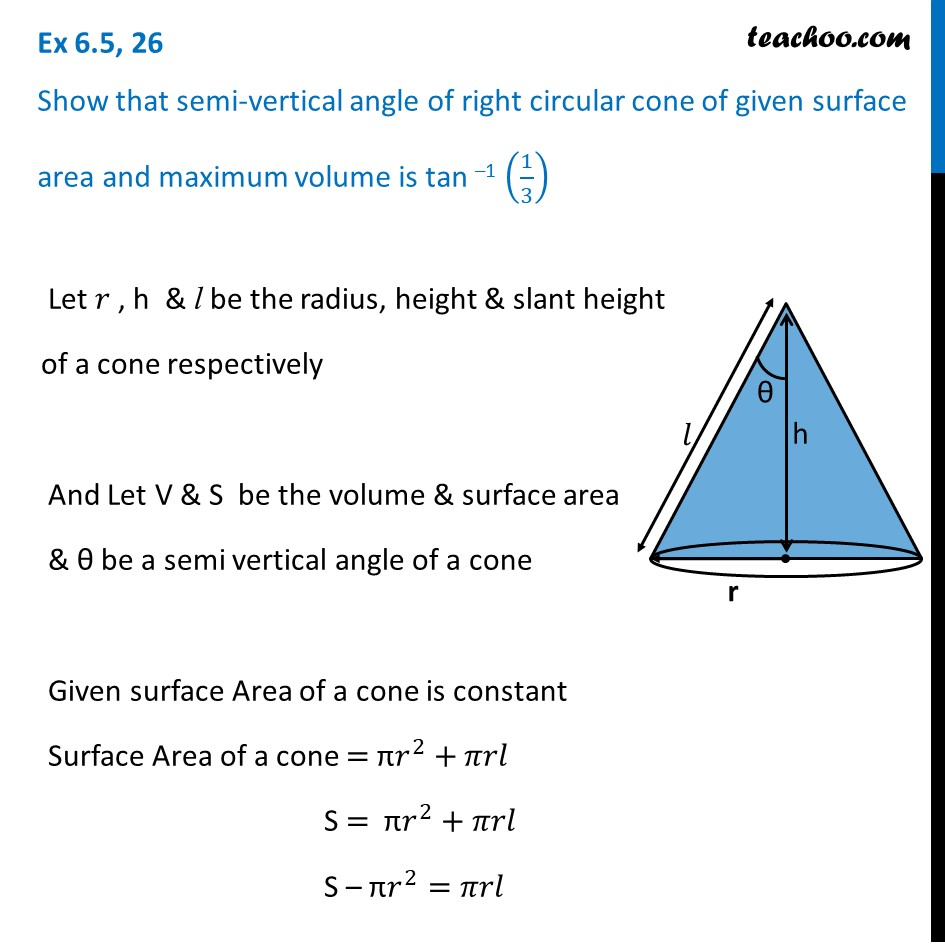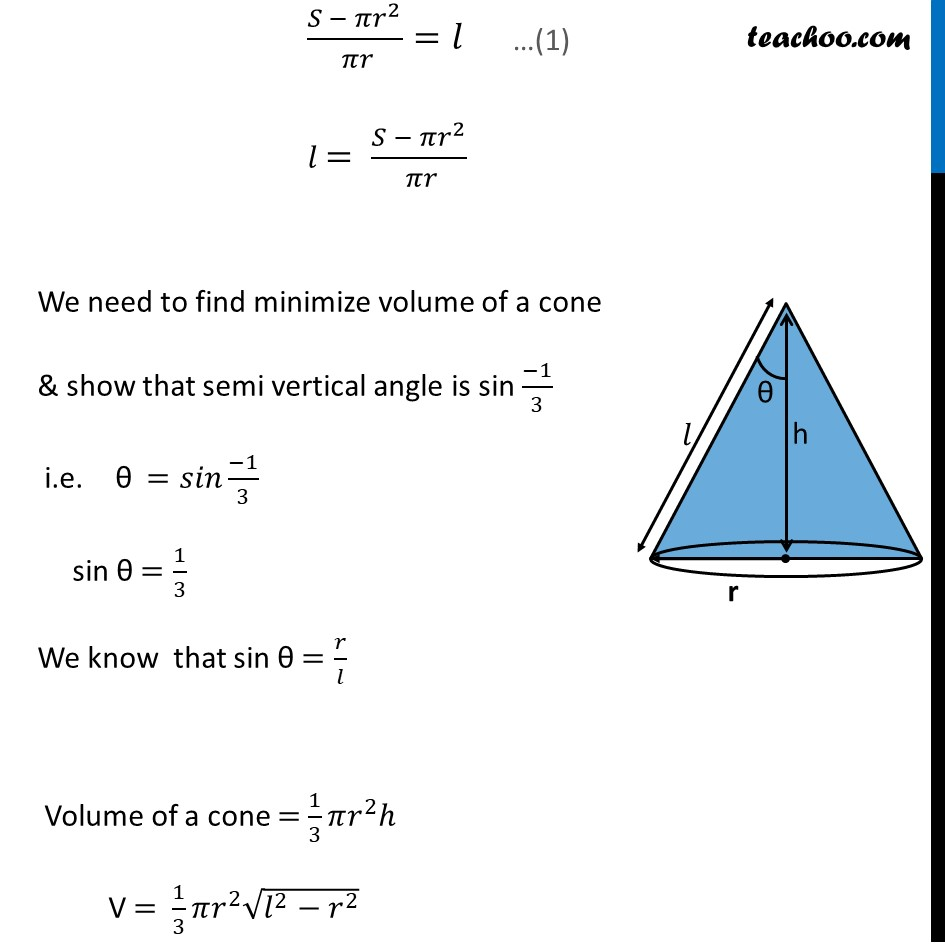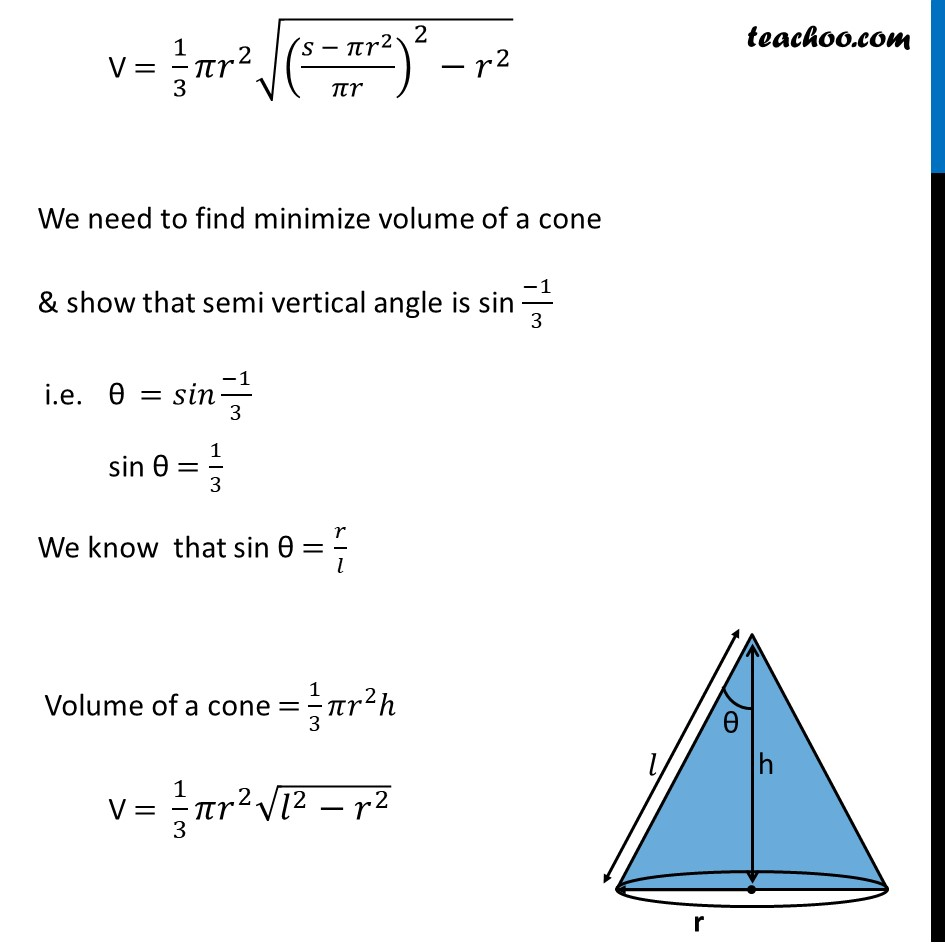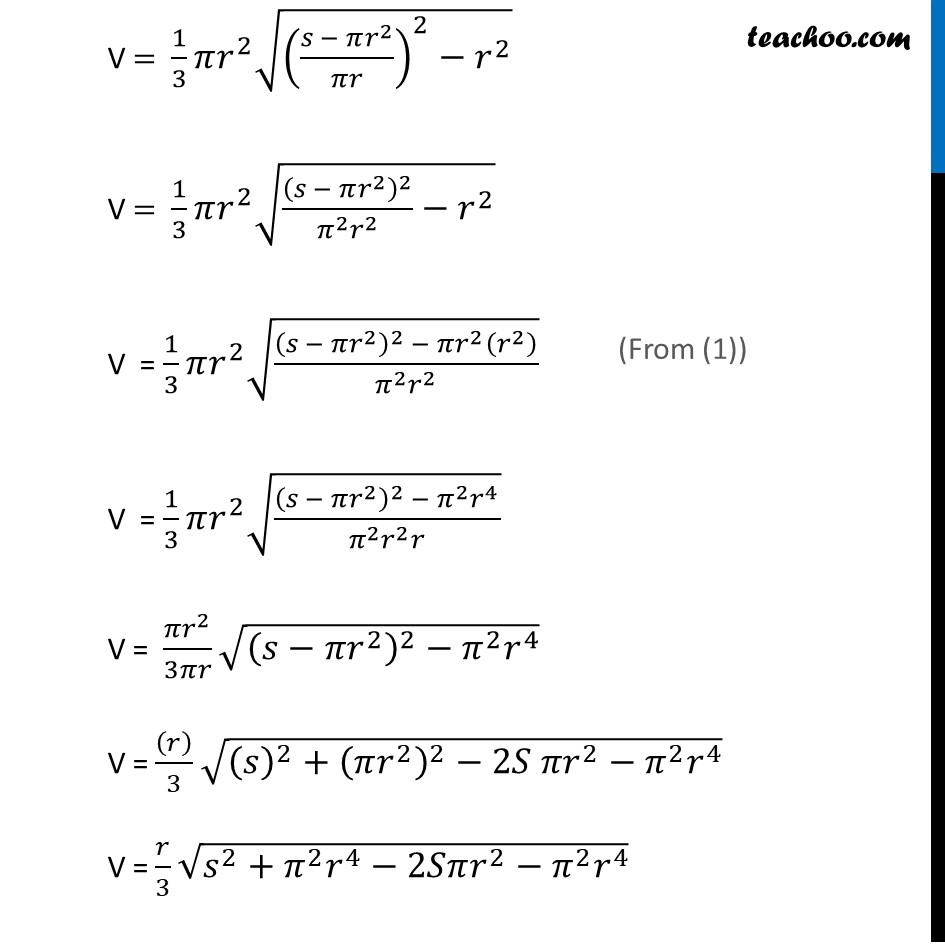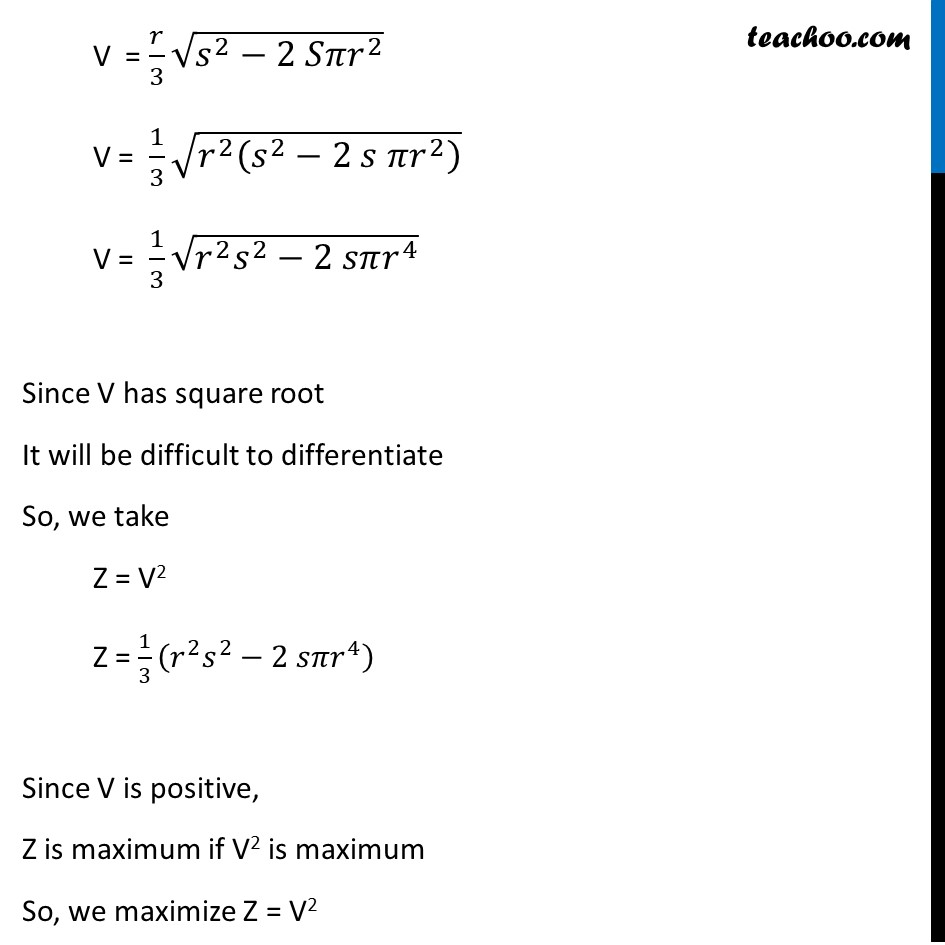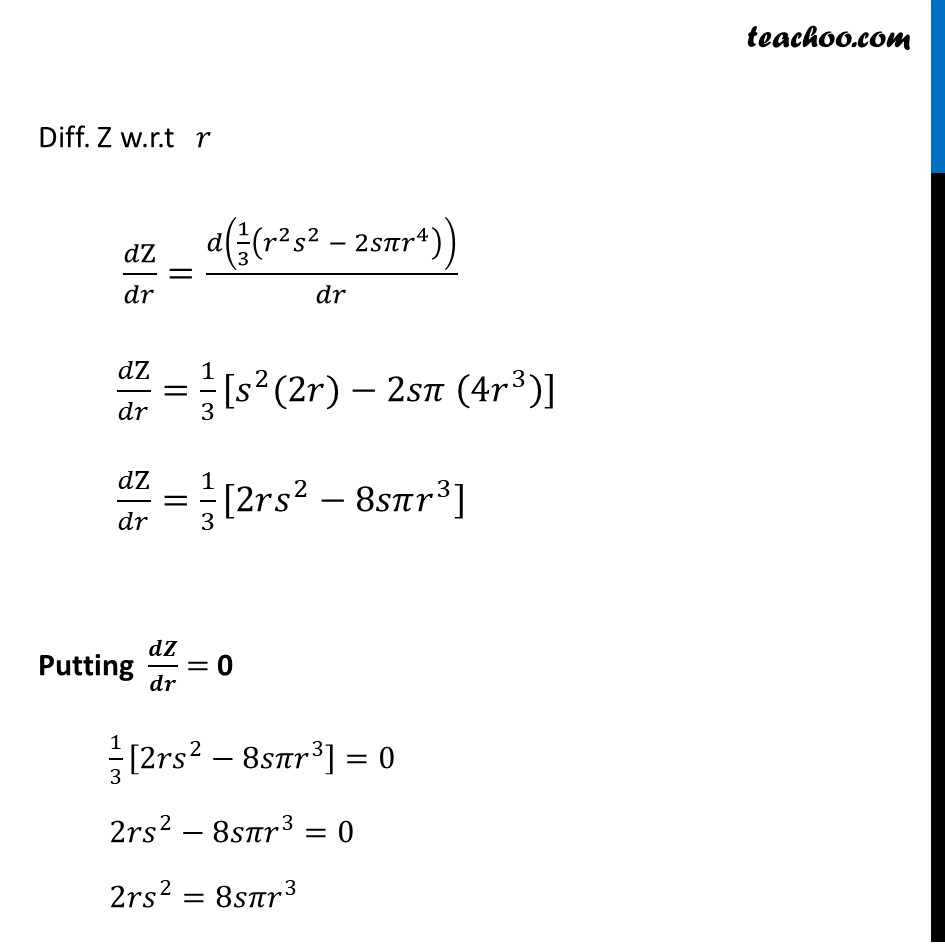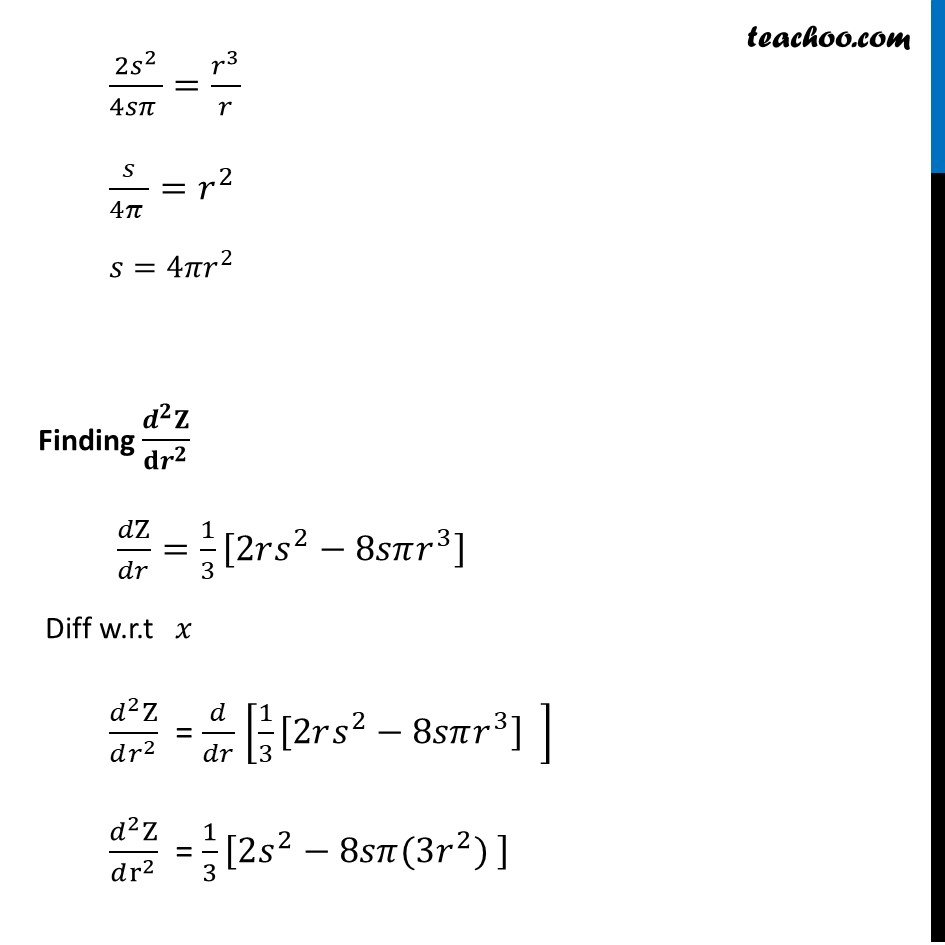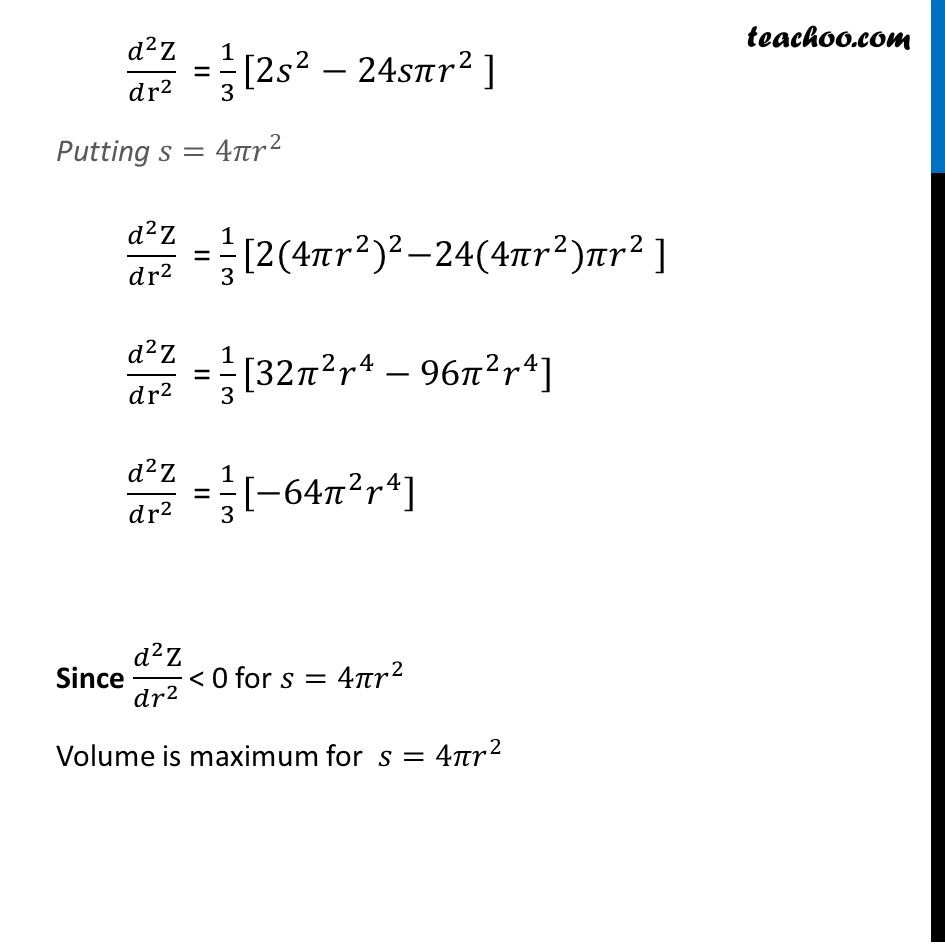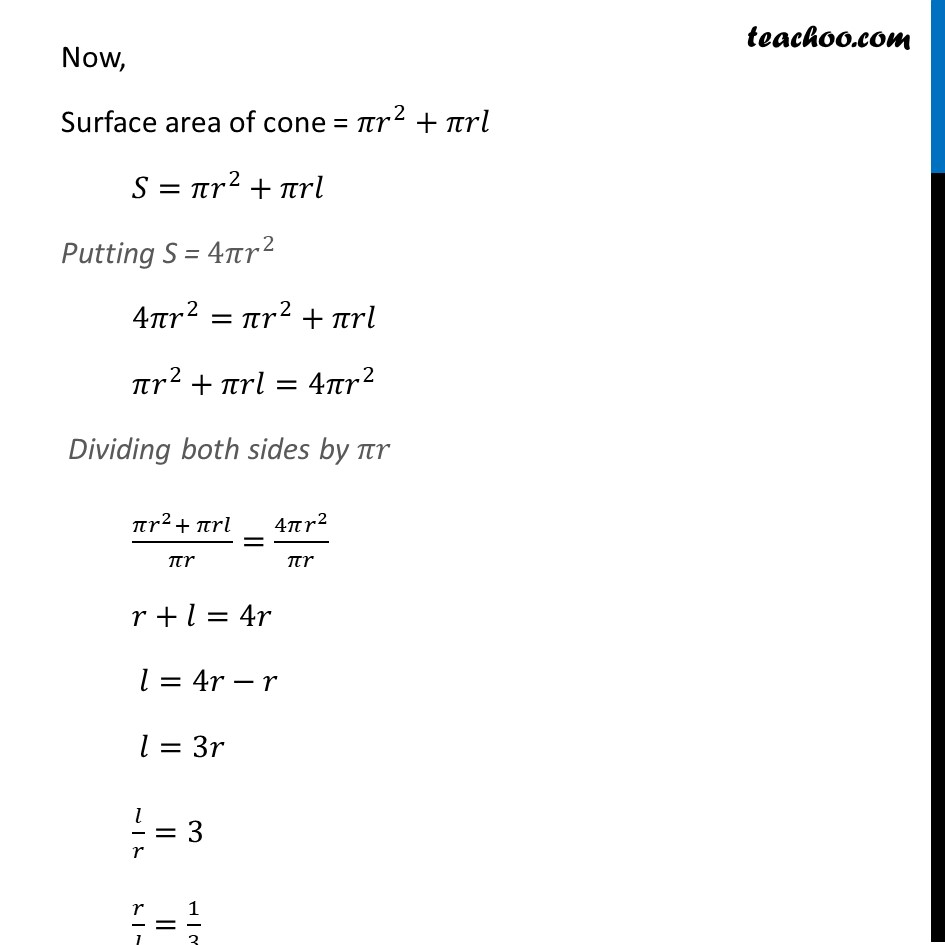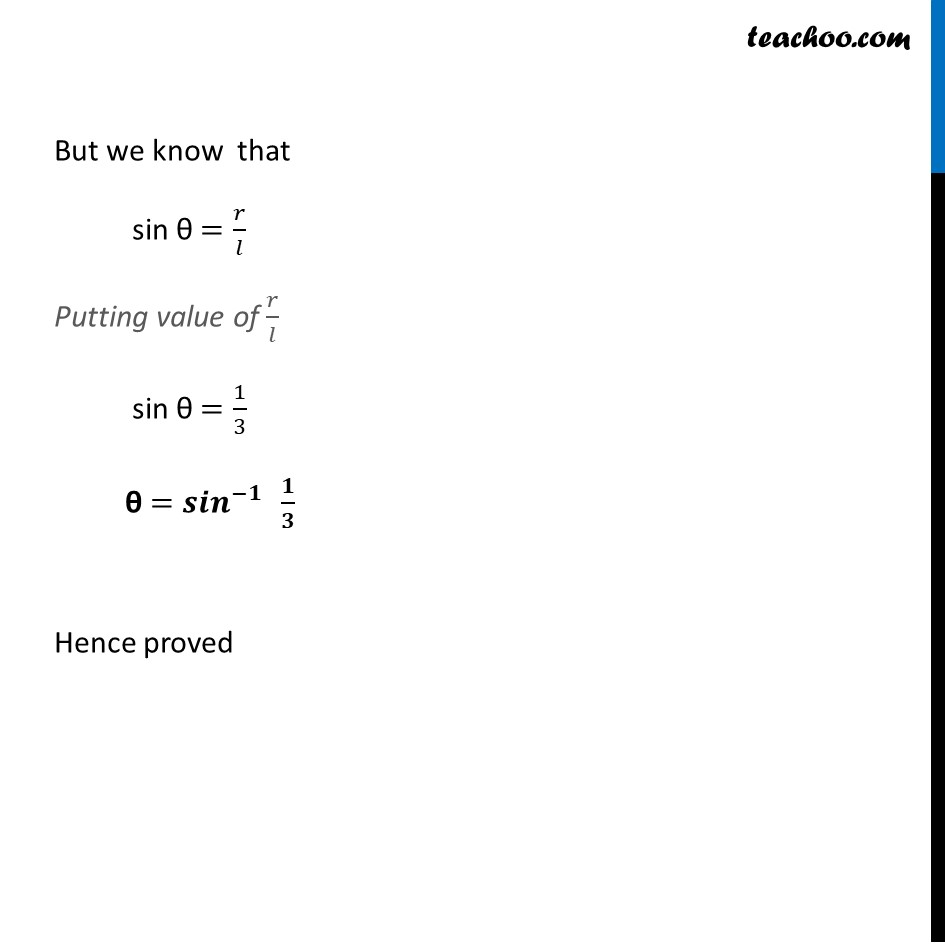### Transcript

Ex 6.5, 26 Show that semi-vertical angle of right circular cone of given surface area and maximum volume is tan –1 (1/3) Let 𝑟 , h & l be the radius, height & slant height of a cone respectively And Let V & S be the volume & surface area & θ be a semi vertical angle of a cone Given surface Area of a cone is constant Surface Area of a cone = π𝑟^2+𝜋𝑟𝑙 S = π𝑟^2+𝜋𝑟𝑙 S – π𝑟^2=𝜋𝑟𝑙 (𝑆 − 𝜋𝑟^2)/(\ 𝜋𝑟)=𝑙 𝑙 = (𝑆 − 𝜋𝑟^2)/(\ 𝜋𝑟) We need to find minimize volume of a cone & show that semi vertical angle is sin (−1)/3 i.e. θ =𝑠𝑖𝑛 (−1)/3 sin θ =1/3 We know that sin θ =𝑟/𝑙 Volume of a cone = 1/3 𝜋𝑟^2 ℎ V = 1/3 𝜋𝑟^2 √(𝑙^2−𝑟^2 ) V = 1/3 𝜋𝑟^2 √(((𝑠 − 𝜋𝑟^2)/𝜋𝑟)^2−𝑟^2 ) We need to find minimize volume of a cone & show that semi vertical angle is sin (−1)/3 i.e. θ =𝑠𝑖𝑛 (−1)/3 sin θ =1/3 We know that sin θ =𝑟/𝑙 Volume of a cone = 1/3 𝜋𝑟^2 ℎ V = 1/3 𝜋𝑟^2 √(𝑙^2−𝑟^2 ) V = 1/3 𝜋𝑟^2 √(((𝑠 − 𝜋𝑟^2)/𝜋𝑟)^2−𝑟^2 ) We need to find minimize volume of a cone & show that semi vertical angle is sin (−1)/3 i.e. θ =𝑠𝑖𝑛 (−1)/3 sin θ =1/3 We know that sin θ =𝑟/𝑙 Volume of a cone = 1/3 𝜋𝑟^2 ℎ V = 1/3 𝜋𝑟^2 √(𝑙^2−𝑟^2 ) V = 1/3 𝜋𝑟^2 √(((𝑠 − 𝜋𝑟^2)/𝜋𝑟)^2−𝑟^2 ) V = 1/3 𝜋𝑟^2 √((𝑠 − 𝜋𝑟^2 )^2/(𝜋^2 𝑟^2 )−𝑟^2 ) V = 1/3 𝜋𝑟^2 √(((𝑠 − 𝜋𝑟^2 )^2 − 𝜋𝑟^2 (𝑟^2 ))/(𝜋^2 𝑟^2 )) V = 1/3 𝜋𝑟^2 √(((𝑠 − 𝜋𝑟^2 )^2 − 𝜋^2 𝑟^4)/(𝜋^2 𝑟^2 𝑟)) V = (𝜋𝑟^2)/3𝜋𝑟 √((𝑠−𝜋𝑟^2 )^2−𝜋^2 𝑟^4 ) V = ((𝑟))/3 √(〖(𝑠)^2+(𝜋𝑟^2 )〗^2−2𝑆 〖𝜋𝑟〗^2−𝜋^2 𝑟^4 ) V = 𝑟/3 √(𝑠^2+𝜋^2 𝑟^4−2𝑆𝜋𝑟^2−𝜋^2 𝑟^4 ) V = 𝑟/3 √(𝑠^2−2 𝑆𝜋𝑟^2 ) V = 1/3 √(𝑟^2 (𝑠^2−2 𝑠 𝜋𝑟^2 ) ) V = 1/3 √(𝑟^2 𝑠^2−2 𝑠𝜋𝑟^4 ) Since V has square root It will be difficult to differentiate So, we take Z = V2 Z = 1/3 (𝑟^2 𝑠^2−2 𝑠𝜋𝑟^4 ) Since V is positive, Z is maximum if V2 is maximum So, we maximize Z = V2 Diff. Z w.r.t 𝑟 𝑑Z/𝑑𝑟=𝑑(1/3 (𝑟^2 𝑠^2 − 2𝑠𝜋𝑟^4 ))/𝑑𝑟 𝑑Z/𝑑𝑟=1/3 [𝑠^2 (2𝑟)−2𝑠𝜋 (4𝑟^3 )] 𝑑Z/𝑑𝑟=1/3 [2𝑟𝑠^2−8𝑠𝜋𝑟^3 ] Putting 𝒅𝒁/𝒅𝒓 = 0 1/3 [2𝑟𝑠^2−8𝑠𝜋𝑟^3 ]=0 2𝑟𝑠^2−8𝑠𝜋𝑟^3=0 2𝑟𝑠^2=8𝑠𝜋𝑟^3 (2𝑠^2)/(4𝑠𝜋 )=𝑟^3/𝑟 𝑠/(4𝜋 )=𝑟^2 𝑠=4𝜋𝑟^2 Finding (𝒅^𝟐 𝐙)/(𝐝𝒓^𝟐 ) 𝑑Z/𝑑𝑟=1/3 [2𝑟𝑠^2−8𝑠𝜋𝑟^3 ] Diff w.r.t 𝑥 (𝑑^2 Z)/(𝑑𝑟^2 ) = 𝑑/𝑑𝑟 [1/3 [2𝑟𝑠^2−8𝑠𝜋𝑟^3 ] " " ] (𝑑^2 Z)/(𝑑r^2 ) = 1/3 [2𝑠^2−8𝑠𝜋(3𝑟^2) ] (𝑑^2 Z)/(𝑑r^2 ) = 1/3 [2𝑠^2−24𝑠𝜋𝑟^2 ] Putting 𝑠=4𝜋𝑟^2 (𝑑^2 Z)/(𝑑r^2 ) = 1/3 [2〖(4𝜋𝑟^2)〗^2−24(4𝜋𝑟^2)𝜋𝑟^2 ] (𝑑^2 Z)/(𝑑r^2 ) = 1/3 [32𝜋^2 𝑟^4−96𝜋^2 𝑟^4 ] (𝑑^2 Z)/(𝑑r^2 ) = 1/3 [−64𝜋^2 𝑟^4 ] Since (𝑑^2 Z)/(𝑑𝑟^2 ) < 0 for 𝑠=4𝜋𝑟^2 Volume is maximum for 𝑠=4𝜋𝑟^2 Now, Surface area of cone = 𝜋𝑟^2+𝜋𝑟𝑙 𝑆=𝜋𝑟^2+𝜋𝑟𝑙 Putting S = 4𝜋𝑟^2 4𝜋𝑟^2=𝜋𝑟^2+𝜋𝑟𝑙 𝜋𝑟^2+𝜋𝑟𝑙=4𝜋𝑟^2 Dividing both sides by 𝜋𝑟 (𝜋𝑟^2+ 𝜋𝑟𝑙)/𝜋𝑟=(4𝜋𝑟^2)/𝜋𝑟 𝑟+𝑙=4𝑟 𝑙=4𝑟−𝑟 𝑙=3𝑟 𝑙/𝑟=3 𝑟/𝑙=1/3 But we know that sin θ =𝑟/𝑙 Putting value of 𝑟/𝑙 sin θ =1/3 θ =〖𝒔𝒊𝒏〗^(−𝟏)⁡ 𝟏/𝟑 Hence proved